# Domain and Range of a Function

Domain and Range of a Function

Functions in mathematics can be compared to the operations of a vending (soda) machine.

When you put in a certain amount of money, you can select different types of sodas.Similarly, for functions, we input different numbers and we get new numbers as the result.

Domain and range are one aspect of functions.

You can use quarters and one-dollar bills to buy a soda.

What will happen if you put in pennies?

The machine will not give you any flavor of the soda.

Hence, the domain represents the inputs we can have here, that is, quarters and one-dollar bills.

No matter what amount you pay, you won't get a cheeseburger from a soda machine.

Thus, range is the possible outputs we can have here, that is, the flavors of soda in the machine.

In this lesson, once you have understood the basics of domain and range definition and domain and range examples in algebra, you will learn how to find the domain and range of a graph, domain and range of trigonometric functions, domain and range of exponential functions, and domain and range equation.

Check out the interactive examples to know more about the lesson and try your hand at solving a few interesting practice questions at the end of the page.

## Lesson Plan

 1 What Do You Mean By Domain of a Function? 2 Tips and Tricks 3 Important Notes 4 Solved Examples 5 Interactive Questions

## What Do You Mean By Domain of a Function?

### Domain Definition

A domain refers to "all the values" that go into a function.

The domain of a function is the set of all possible inputs for the function.

Consider this box as a function $$f(x)=x^2$$.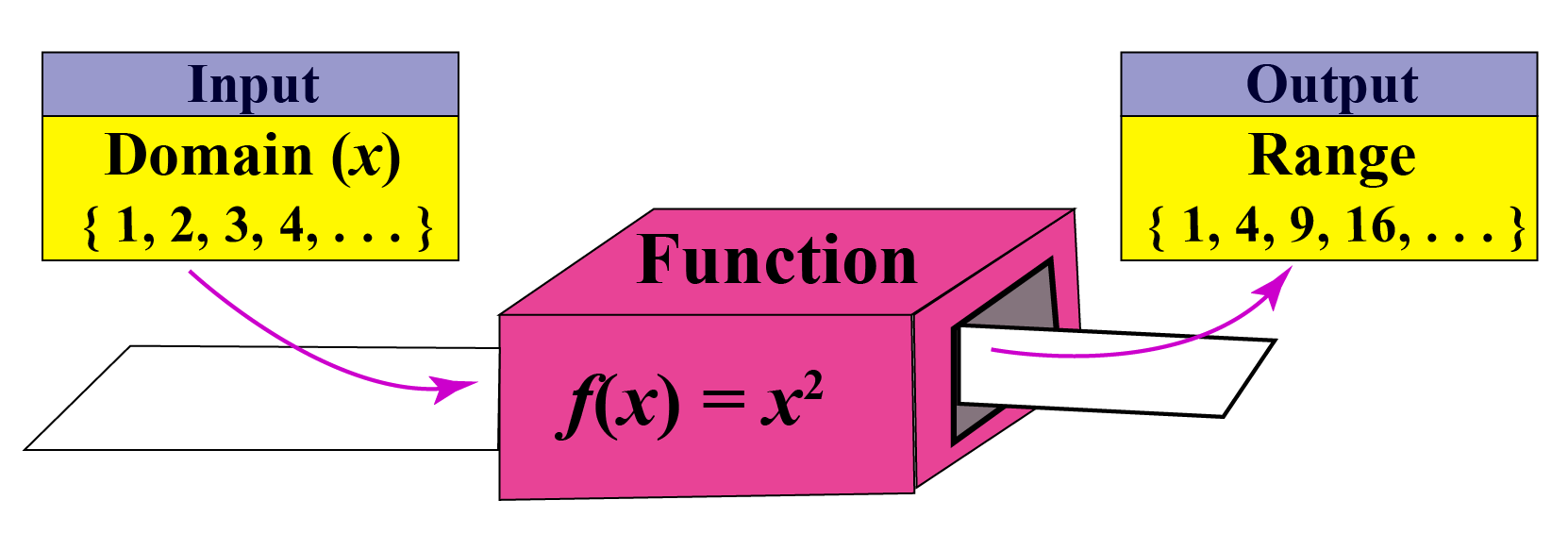Example:

When the function $$f(x)=x^2$$ is given and the values $$x = {1,2,3,4,...}$$, then the domain is simply the set of natural numbers and the output values are called the range.

 $$\text {Domain} → \text {Function} → \text {Range}$$

## What Do You Mean By Range of a Function?

### Range Definition

The range of a function is the set of all its outputs.

Example:

Let us consider the function $$f:\,\, A \rightarrow A$$, where $$A= \{1,2,3,4\}$$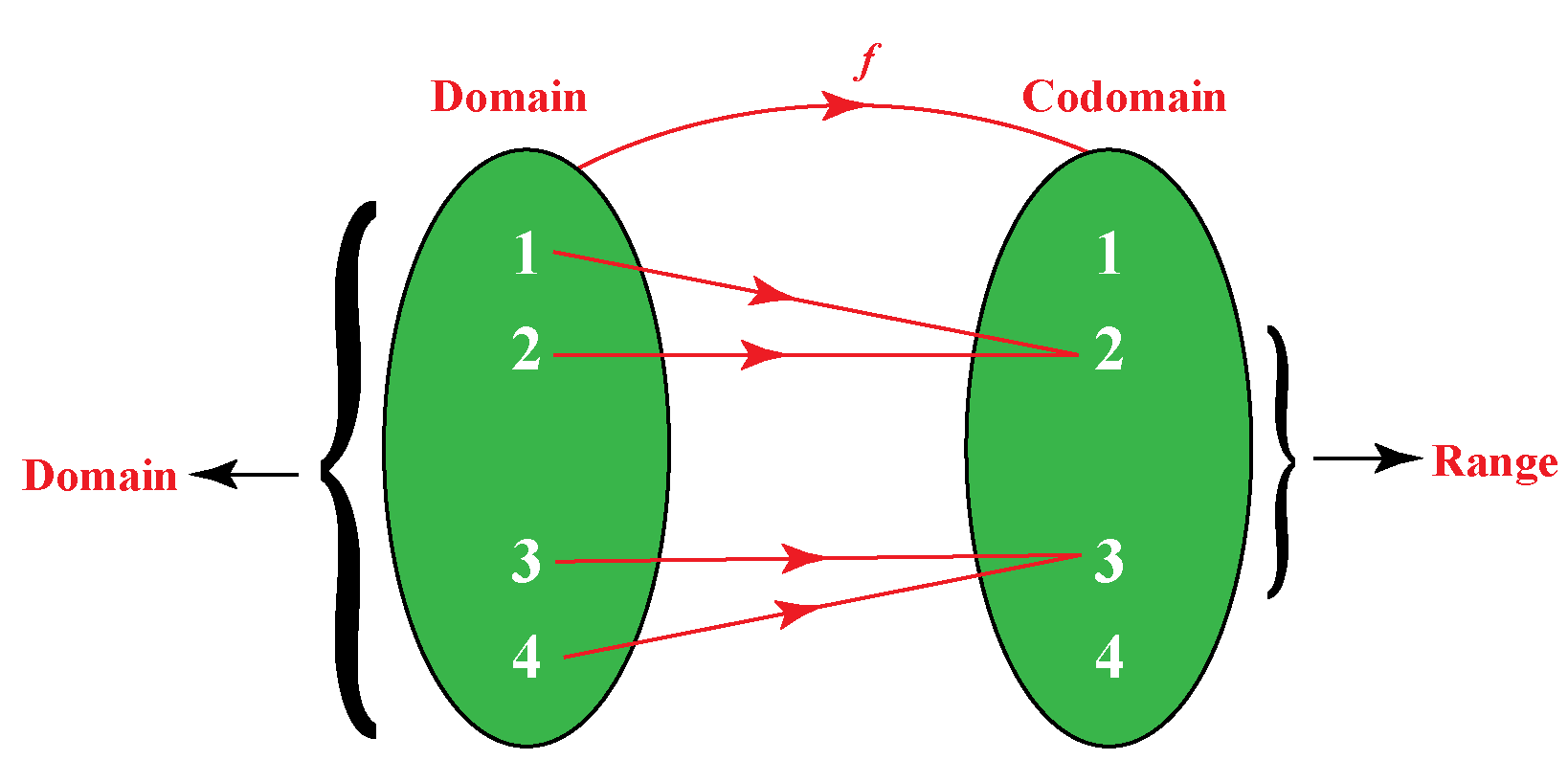The elements of the domain are called pre-images and the elements of the codomain which are mapped are called the images.

Here, the range of the function $$f$$ is the set of all images of the elements of the domain (or) the set of all the outputs of the function.

Thus, the range of $$f$$ = $$\{2, 3\}$$

## How To Find Domain and Range of a Function?

Let's understand the domain and range of some special functions through examples.

### Domain and Range of Exponential Functions

The function $$y=a^x, a\geq 0$$ is defined for all real numbers.

Hence, the domain of the exponential function is the entire real line.

The exponential function always results in a positive value.

Thus, the range of the exponential function is of the form $$y= |ax+b|$$ is $$\{y \in \mathbb{R}| y > 0\}$$.

 Domain = $$\mathbb{R}$$, Range = $$(0, \infty)$$

Example: $$2^x$$

Look at the graph of this function shown below.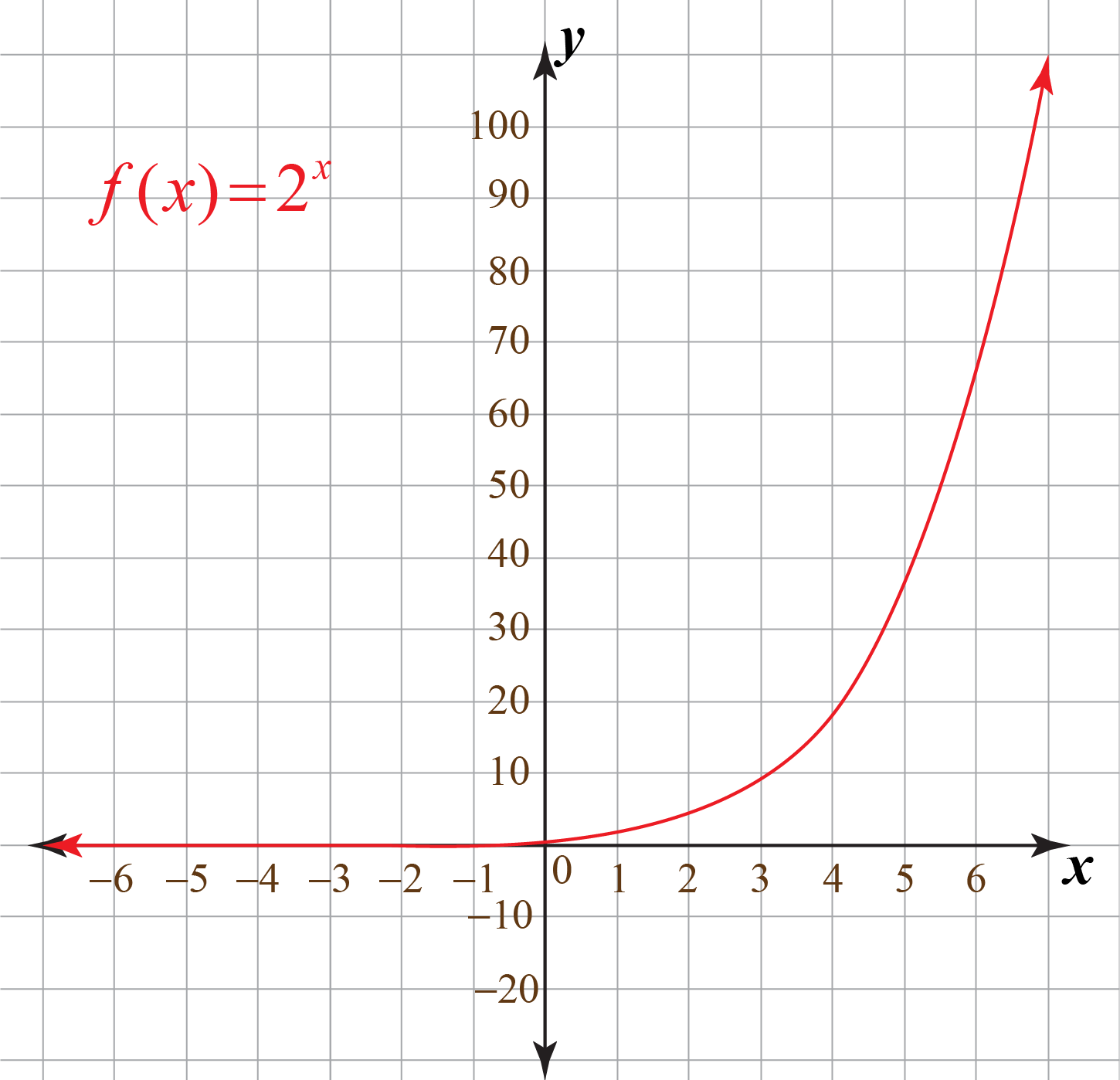Observe that the value of the function is closer to 0 as $$x$$ tends to $$-\infty$$, but it will never attain the value 0

Domain

The domain of the function is the set $$\mathbb{R}$$.

Range

The exponential function always results in positive real values.

### Domain and Range of Trigonometric Functions

Look at the graph of the sine function and cosine function.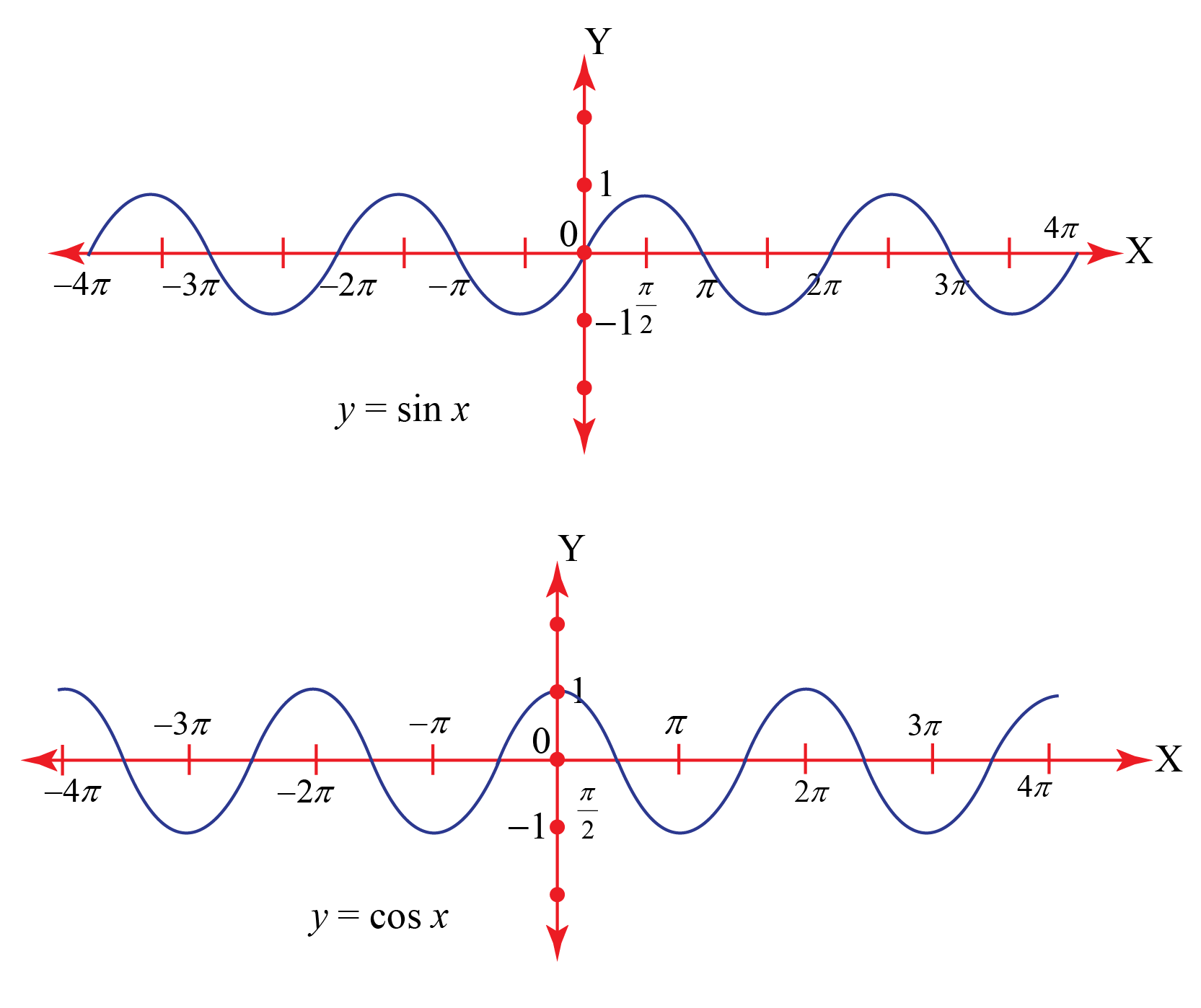What is common in both graphs?

Notice that the value of the functions oscillates between -1 and 1 and it is defined for all real numbers.

Domain

The domain of the functions is the set $$\mathbb{R}$$.

Range

The range of the functions is $$[-1, 1]$$.

### Domain and Range of an Absolute Value Function

The function $$y=|ax+b|$$ is defined for all real numbers.

So, the domain of the absolute value function is the set of all real numbers.

The absolute value of a number always results in a non-negative value.

Thus, the range of an absolute value function of the form $$y= |ax+b|$$ is $$\{y \in \mathbb{R}| y \geq 0\}$$.

 Domain = $$\mathbb{R}$$, Range = $$[0, \infty)$$

Example: $$|6-x|$$

Domain

The domain of the function is the set $$\mathbb{R}$$.

Range

We already know that the absolute value function results in a non-negative value always.

\begin{align} |6-x| &\geq 0\\6-x &\geq 0\\x&\leq 6\end{align}

### Domain and Range of a Square Root Function

The function $$y= \sqrt{ax+b}$$ is defined only for $$x\geq -\dfrac{b}{a}$$.

So, the domain of the square root function is the set of all real numbers greater than or equal to $$\dfrac{b}{a}$$.

We know that the square root of something always results in a non-negative value.

Thus, the range of a square root function is the set of all non-negative real numbers.

 Domain = $$\left[-\dfrac{b}{a}, \infty\right)$$, Range = $$[0, \infty)$$

Example: $$y= 2-\sqrt{-3x+2}$$

Domain

A square root function is defined only when the value inside it is a non-negative number. So for domain,

\begin{align} -3x+2 & \geq 0 \\[0.2cm] -3x & \geq -2\\[0.2cm] x &\leq \dfrac{2}{3} \end{align}

Range

We already know that the square root function results in a non-negative value always.

$\sqrt{-3x+2} \geq 0\\[0.2cm] \text{Multiply -1 on both sides}\\[0.2cm] -\sqrt{-3x+2}\ \leq 0 \\[0.2cm] \text{Adding 2 on both sides},\\[0.2cm] 2-\sqrt{-3x+2}\ \leq 2\\[0.2cm] y \leq 2$

Here are a few tips and tricks for you that will help you find the domain and range of a function easily.Tips and Tricks
1. The easiest method to find the range of a function is by graphing it and looking for the $$y$$-values covered by the graph.
2. In the case of a square root function (or) an absolute value function, the range is always $$y \geq 0$$
3. To find the range of a quadratic function, it is sufficient to see if it has a maximum or minimum value.
The maximum/minimum value of a quadratic function is the y-coordinate of its vertex.
4. To find the domain of the rational function, set the denominator as 0 and solve for the variable.

## Graphs of Domain and Range of Functions

Another way to identify the domain and range of functions is by using graphs.

The domain refers to the set of possible input values.

The domain of a graph consists of all the input values shown on the $$x$$-axis.

The range is the set of possible output values shown on the $$y$$-axis.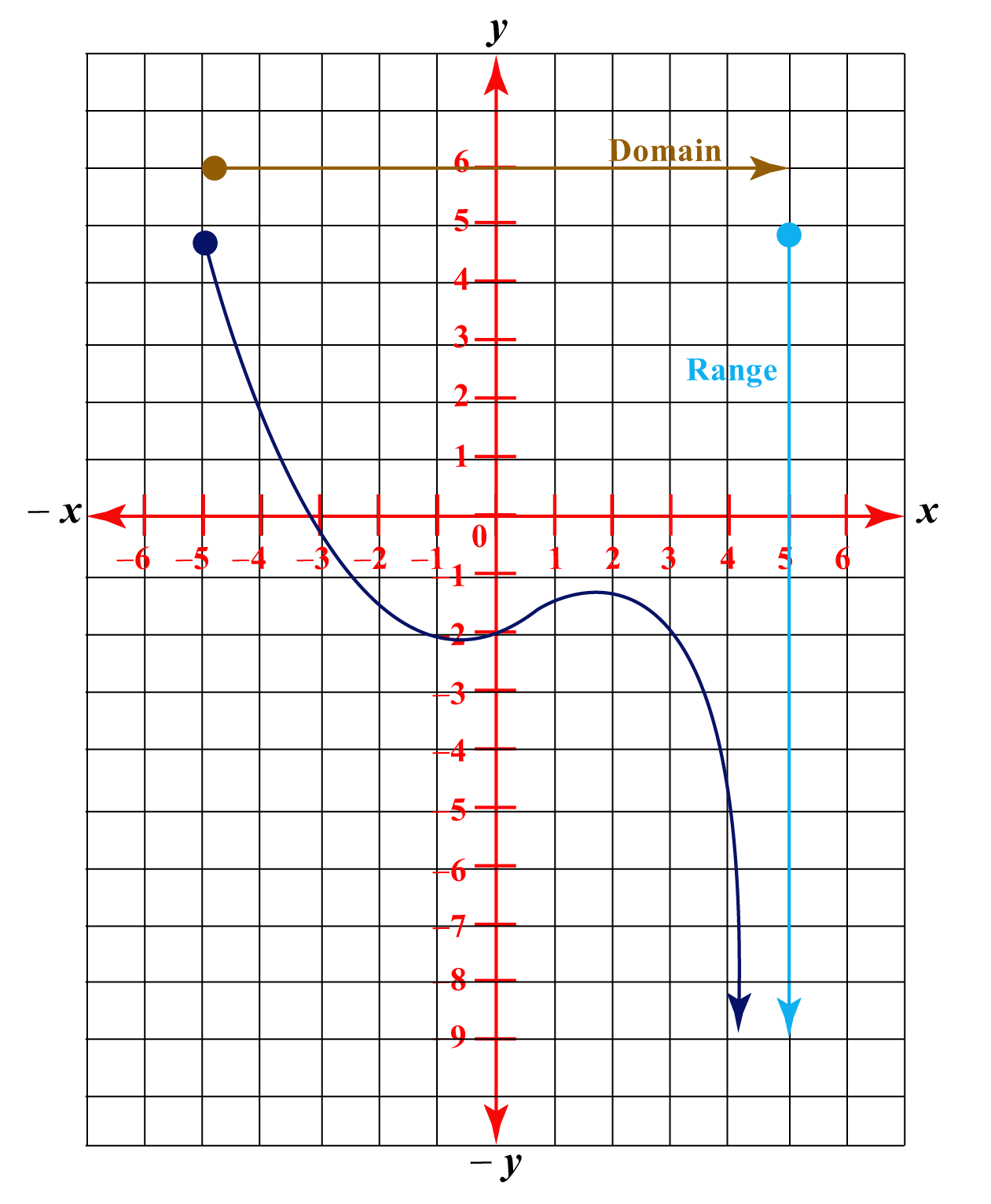### Domain and Range Calculator

Let us see the domain and the range of some different types of functions.

## Domain and Range Examples

 Example 1

Find the range and domain of the function $$f(x) =x^2$$ using the graph of the function shown below.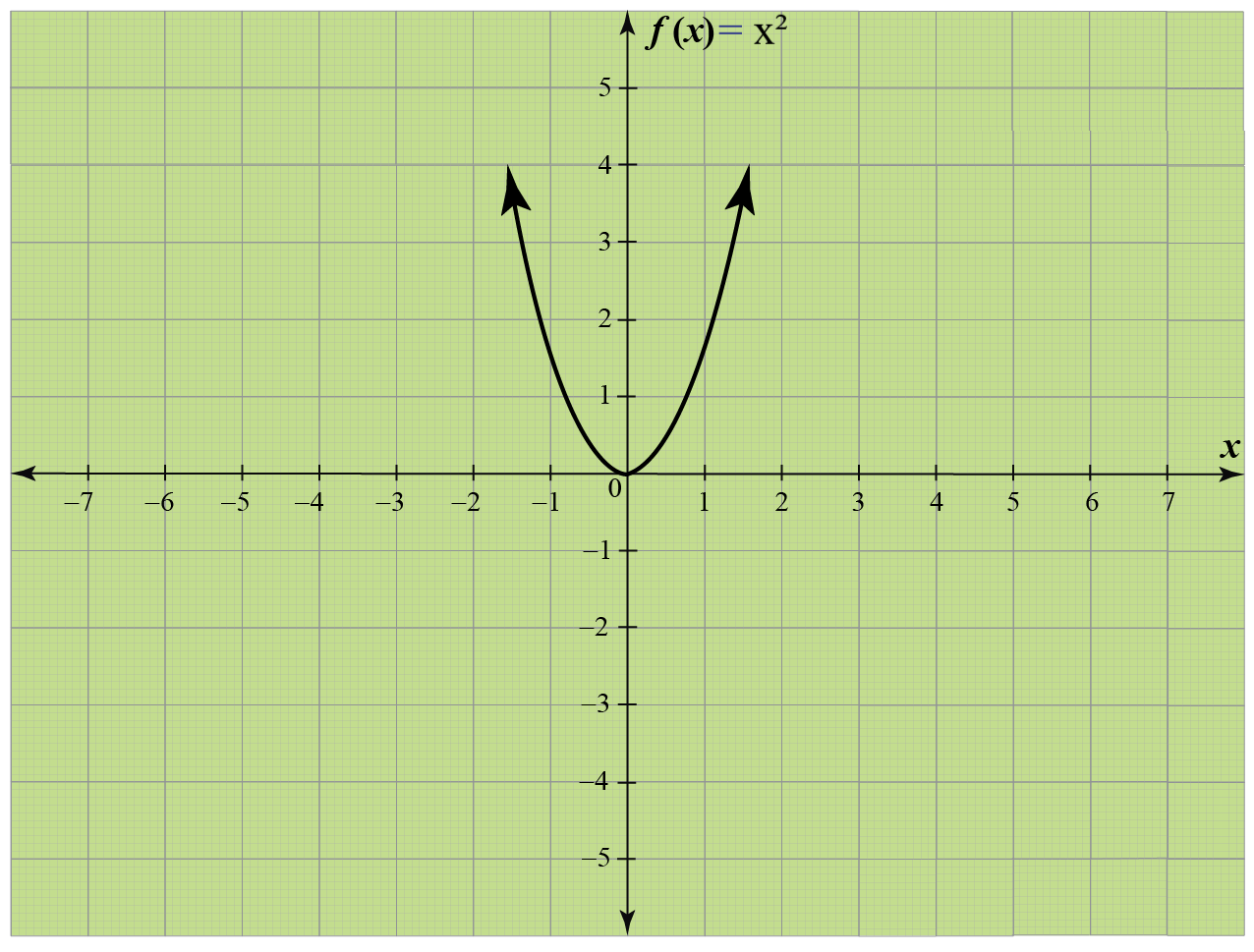Solution

We observe from the graph that the horizontal extent of the graph is from $$( -\infty, \infty)$$.

So, the domain is $$( -\infty, \infty)$$.

Now, the vertical extent of the graph goes from $$0$$ to $$\infty$$.

So, the range is  $$( 0, \infty)$$.

Thus, for the quadratic function $$f(x) =x^2$$, the domain is the set of all real numbers and the range is only non-negative real numbers.

 Domain = $$( -\infty, \infty)$$ and Range = $$( 0, \infty)$$
 Example 2

We define a function $$f: \mathbb{R}-{0} \rightarrow \mathbb{R}$$ as $$f(x)=\dfrac{1}{x}$$.

Complete the table shown below.

$$x$$ -2 -1.5 -1 -0.5 0.25 0.5 1 1.5 2
$$y=\dfrac{1}{x}$$

Find the domain and range of the function.

Solution

Let's complete the given table by finding the values of the function at the given values $$x$$.

$$x$$ -2 -1.5 -1 -0.5 0.25 0.5 1 1.5 2
$$y=\dfrac{1}{x}$$ -0.5 -0.67 -1 -2 4 2 1 0.67 0.5

Let's draw the graph of the function.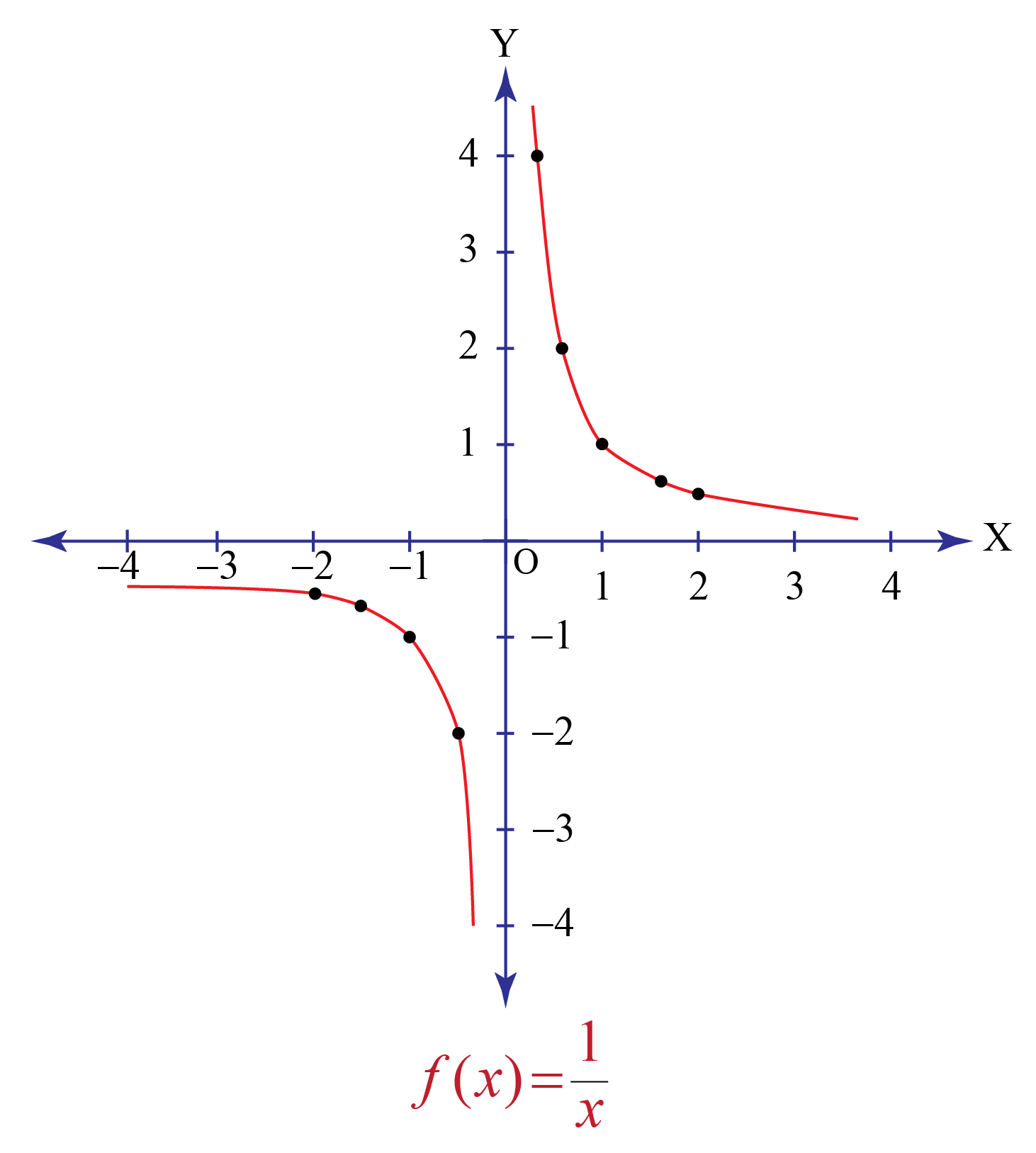From the graph, we can observe that the domain and the range of the function are all real numbers except 0.

 So, the domain and the range of $$f(x)=\dfrac{1}{x}$$ is $$\mathbb{R}/\{0\}$$.
 Example 3

Ms. Amy asked her students to find the range and domain of the function given on the board.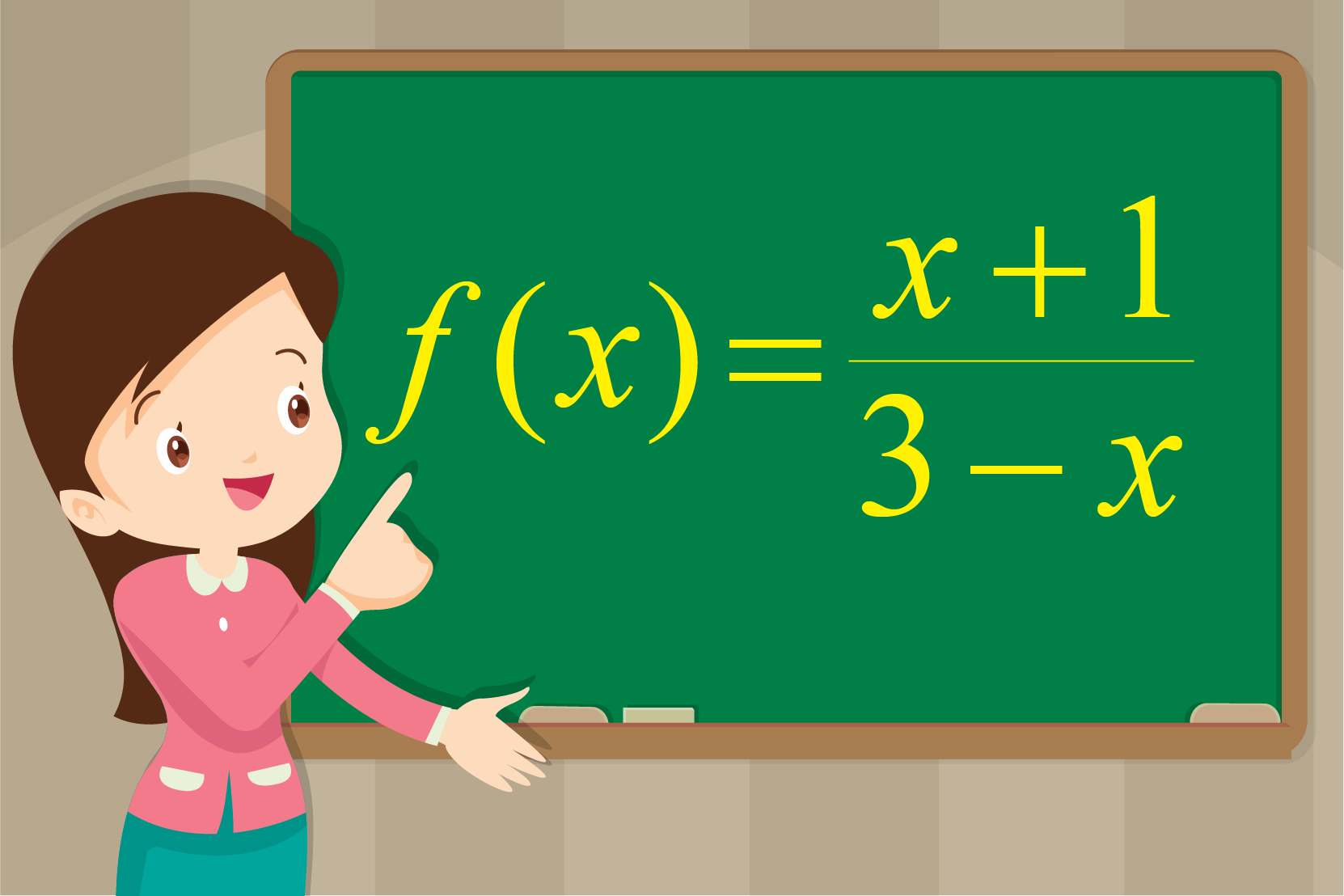Can you determine the same?

Solution

At first, we will set the denominator equal to 0, and then we will solve for $$x$$.

\begin{align*} 3 -x&= 0 \\ -x&= -3 \\ x &= 3 \end{align*}

Hence, we will exclude $$3$$ from the domain.

So, the domain is the set of real numbers $$x$$ where $$( x< 3)$$ and $$(x> 3 )$$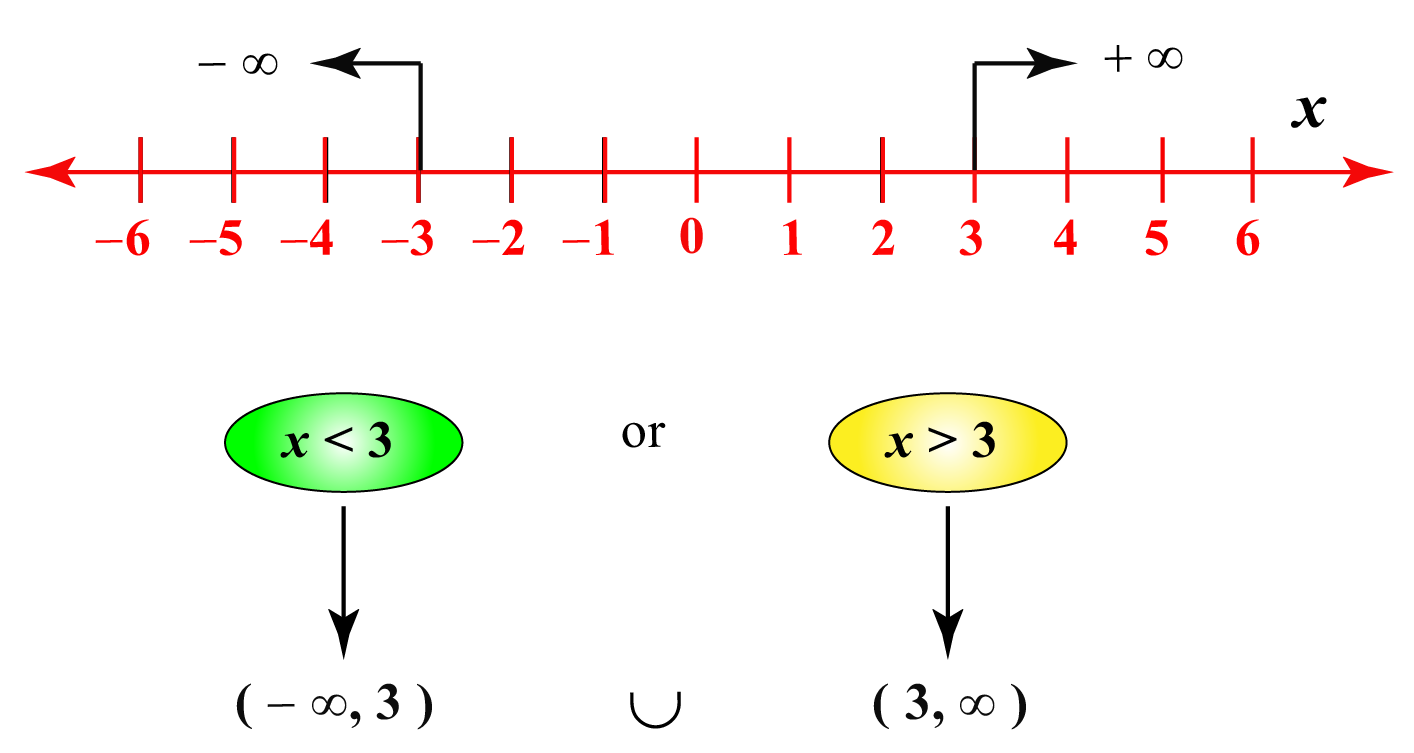Let's find the range of $$y=\dfrac{x+1}{3-x}$$

Let us solve the given equation for $$x$$

\begin{align} (3-x)y &= x+1\\[0.2cm] 3y-xy &=x+1\\[0.2cm] 3y-1&=x+xy\\[0.2cm] x(1+y) &= 3y-1\\[0.2cm] x &= \dfrac{3y-1}{1+y}\end{align}

The final equation is a fraction and a fraction is NOT defined when its denominator is zero. So

\begin{align}1+y &\neq 0 \\[0.2cm] y &\neq-1 \end{align}

Therefore, the range of the given function is the set of all real number excluding -1

 Domain = $$(-\infty,3) \cup (3, \infty)$$, Range = $$(-\infty,-1) \cup (-1, \infty)$$

## Interactive Questions

Here are a few activities for you to practice.

Select/type your answer and click the "Check Answer" button to see the result.

## Let's Summarize

This mini-lesson targeted the fascinating concept of Domain and Range of a Function. The math journey around Domain and Range of a Function starts with what a student already knows, and goes on to creatively crafting a fresh concept in the young minds. Done in a way that is not only relatable and easy to grasp but will also stay with them forever. Here lies the magic with Cuemath.

We hope you enjoyed learning about the domain and range definition, domain and range of a graph, domain and range equation, domain and range of trigonometric functions, domain and range of exponential function along with domain and range examples.

## About Cuemath

At Cuemath, our team of math experts is dedicated to making learning fun for our favorite readers, the students!

Through an interactive and engaging learning-teaching-learning approach, the teachers explore all angles of a topic.

Be it worksheets, online classes, doubt sessions, or any other form of relation, it’s the logical thinking and smart learning approach that we, at Cuemath, believe in.

## FAQs on Domain and Range of a Function

### 1. What is the range in a function?

The range of a function is the set of all the outputs a function can give.

### 2. How do you write the domain and range?

We write the domain of a function by finding the set of all possible inputs for the function.

We write the range of a function by finding the set of all the outputs a function can give.

### 3. What is the natural domain and range of a function?

The natural domain of a function is the set of all possible inputs for the function.

The range of a function is the set of all the outputs a function can give.

### 4. What is the domain and range of a constant function?

Let the constant function be $$f(x)=k$$.

The domain of a constant function is given by $$\mathbb{R}$$, that is, the set of real numbers.

The range of a constant function is given by the singleton set, $${k}$$.

### 5. How to find the domain and range of a function algebraically?

Let the function be $$y=f(x)$$.

To calculate the domain of the function algebraically, we simply solve the equation to determine the values of the independent variable $$x$$.

To calculate the range of the function algebraically, we simply express $$x$$ as $$x=g(y)$$ and then find the domain of $$g(y)$$.

### 6. How to find the domain and range of an equation?

To find the domain, we simply solve the equation $$y=f(x)$$ to determine the values of the independent variable $$x$$.

To calculate the range of the function, we simply express $$x$$ as $$x=g(y)$$ and then find the domain of $$g(y)$$.

### 7. What is the difference between domain and range of a function?

The domain of a function is the set of all possible inputs for the function.

The range of a function is the set of all the outputs a function can give.

### 8. What is the domain and range of a relation?

Let $$R$$ be the relation from a non-empty set $$A$$ to a non-empty set $$B$$.

The set of first elements in the order pairs in relation $$R$$ is called the domain.

The set of second elements in the order pairs in relation $$R$$ is called the range.

### 9. What is the domain and range of composite functions?

Let the composite function be $$h=f \circ g$$.

The domain of $$h$$ is either same as $$f$$ or lies within the domain of $$f$$.

The range $$h$$ must lie with the range of $$g$$.

### 10. What is the range of a quadratic function?

The range of a quadratic function $$y=a(x-h)^2+k$$ is:

• $$y \geq k$$ if the function has a minimum value, that is, when a>0
• $$y\leq k$$ if the function has a maximum value, that is, when a<0

Download Functions Worksheets
Functions
grade 10 | Answers Set 1
Functions
grade 10 | Questions Set 2
Functions
grade 10 | Answers Set 2
Functions
grade 10 | Questions Set 1
More Important Topics
Numbers
Algebra
Geometry
Measurement
Money
Data
Trigonometry
Calculus